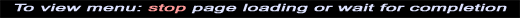Below, we will demonstrate a method to calculate the theoretical thrust that that a propeller or rotor can generate. Of course in a helicopter, the rotor disk is oriented such that we call its force "lift" rather than thrust, "thrust" would be used in the case of an airplane. On a helicopter, the force of a tail rotor would be best described as thrust.

The first step is to measure the diameter of the rotor or propeller and calculate the area in square feet. Area is defined as:

A [ft^2] = Pi * r^2

-or-

A [ft^2] = (Pi/4) * D^2

where r is the radius of the propeller or rotor disk and D is the diameter in feet, of course Pi is the number 3.141592653589793238462643

If we know the area of the disk in square feet, we then need to know the amount of power that is delivered to the rotor. This needs to be in the units of horsepower. To convert the metric system of units Watts to horsepower, use the conversion: 1 horsepower = 745.699872 Watts. The goal is to calculate a parameter called "power loading" in units of horsepower per square foot. Power loading is calculated by:

PL [hp/ft^2] = power / A

where "power" is the power delivered to the rotor or propeller and A is the area, calculated above. This is very important---approximately 10 to 15 % of the engine's power will be delivered to the tail rotor to counteract torque. This number obvisouly varies but 10 to 15 percent is a good starting point. If you have a 100 horsepower engine in your helicopter, expect only 85 to 90 horsepower to actually get to the main rotor. Additionally, you would need to reduce the power by an additional small percentage to account for frictional losses in the drive system. In the case of a tandem rotor helicopter such as the Chinook or meshed rotor like the Kmax, all of the power will be delivered to the main rotor and this in fact is the reason those helicopters are so well suited for heavy load lifting operations.

Using the parameter PL [hp/ft^2], we use an empiracly defined formula to calculate the thrust loading (after McCormick). Thrust loading is in the units of pound per horsepower and is a function of power and rotor disk area. Thrust loading (TL) is calculated:

TL [lb/hp]= 8.6859 * PL^(-0.3107)

Now that thrust loading is calculated, we can find the total thrust of the propeller (or lift of the rotor).

Lift = TL * power >>>[lb] = [lb/hp] * [hp]
*note: Calculation results are in pounds-thrust, NOT pounds-mass

Below are some common examples of the thrust developed by common aircraft engines and typical helicopters:

Theoretical thrust developed by common airplane engine - propeller combinations:
300 hp, 78" propeller develops 1,300 pounds of thrust
80 hp, 50" propeller develops 400 pounds of thrust
1.5 hp, 12" propeller develops 10.5 pounds of thrust

Theoretical lift developed by common helicopters:
300 hp, 30' rotor develops 3,400 pounds of lift
(neglecting loss to tail rotor of 10-15%)
25 hp, 12' rotor develops 347 pounds of lift
(neglecting loss to tail rotor of 10-15%)
2 hp, 6' rotor develops 39 pounds of lift
(neglecting loss to tail rotor of 10-15%)
0.25 hp, 10.5" tail-rotor develops 2.9 pounds of thrust

Full series of example calculations:

[EQ1]: PL [hp/ft^2] = power / A
[EQ2]: TL [lb/hp]= 8.6859 * PL^(-0.3107)
[EQ3]:
Lift = TL * power >>>[lb] = [lb/hp] * [hp]

Using equation one, we calculate power loading (PL) of a 6 foot diameter (72") disk with 300hp absorbed.
PL = 300 / (pi*3'^2)
PL = 300 /
28.27
PL = 10.61 hp/ft^2

Using equation two, we calculate the thrust loading. Typical communication/interpretation error is in the negative exponent of the equation. Remember, X^(-Y) is the same as 1/(X^Y).
TL = 8.6859 * (10.61^-.3107)
TL = 8.6859 / (10.61^.3107)

TL = 8.6859 / 2.083
TL = 4.2 [lb/hp]

Using equation three, we calculate the lift/thrust.
Lift = TL * power
Lift = 4.2 * 300
Lift = 1,251 pounds